Test: Parallel Lines And Co-Interior Angles

# Test: Parallel Lines And Co-Interior Angles - Class 9

Test Description

## 15 Questions MCQ Test Mathematics (Maths) Class 9 - Test: Parallel Lines And Co-Interior Angles

Test: Parallel Lines And Co-Interior Angles for Class 9 2023 is part of Mathematics (Maths) Class 9 preparation. The Test: Parallel Lines And Co-Interior Angles questions and answers have been prepared according to the Class 9 exam syllabus.The Test: Parallel Lines And Co-Interior Angles MCQs are made for Class 9 2023 Exam. Find important definitions, questions, notes, meanings, examples, exercises, MCQs and online tests for Test: Parallel Lines And Co-Interior Angles below.
Solutions of Test: Parallel Lines And Co-Interior Angles questions in English are available as part of our Mathematics (Maths) Class 9 for Class 9 & Test: Parallel Lines And Co-Interior Angles solutions in Hindi for Mathematics (Maths) Class 9 course. Download more important topics, notes, lectures and mock test series for Class 9 Exam by signing up for free. Attempt Test: Parallel Lines And Co-Interior Angles | 15 questions in 15 minutes | Mock test for Class 9 preparation | Free important questions MCQ to study Mathematics (Maths) Class 9 for Class 9 Exam | Download free PDF with solutions
 1 Crore+ students have signed up on EduRev. Have you?
Test: Parallel Lines And Co-Interior Angles - Question 1

### From figure, identify the incorrect statement, given that l || m and t is transversal.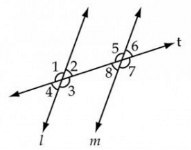Detailed Solution for Test: Parallel Lines And Co-Interior Angles - Question 1

Angle 2 and 8 are a pair of alternate interior angles, and thus are equal. so they don't form a pair of supplementary angles.

Test: Parallel Lines And Co-Interior Angles - Question 2

### If two parallel lines are intersected by a transversal, then the bisectors of any two corresponding angles are

Test: Parallel Lines And Co-Interior Angles - Question 3

### In figure, PQ||RS QPO = 70o ROT = 20o. Find the value of x.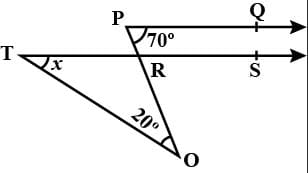Test: Parallel Lines And Co-Interior Angles - Question 4

Given that AB || CD, intersected by a transversal. ∠1 = (120 -x)° and ∠5 = 5x°, then the measures of ∠1 and ∠5 are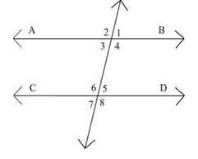Detailed Solution for Test: Parallel Lines And Co-Interior Angles - Question 4
Angle1=angle5(corresponding angles)
therefore,
120-x=5x
or,120=5x+x
or,6x=120
or, x=120/6
or,x=20
and, 120-x=120-20=100
and, 5x=5*20=100

hence proved
Test: Parallel Lines And Co-Interior Angles - Question 5

l, m and n are parallel lines intersected by a transversal p at X, Y and Z respectively. The value of ∠2 will be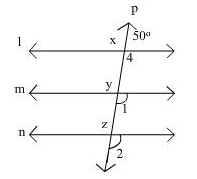Test: Parallel Lines And Co-Interior Angles - Question 6

If AB || CD, CD || EF and y:z = 3:7 then x is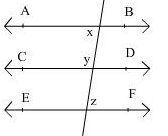Detailed Solution for Test: Parallel Lines And Co-Interior Angles - Question 6

It is given that,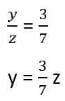Then ∠CON = p

Now CD║EF

So, p = z  (alternate interior angle) .. (1)

Also, p + y = 180°  (linear pair)

y + z = 180° [ from (1) ]

Put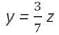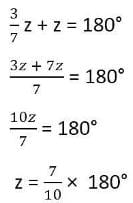z = 126°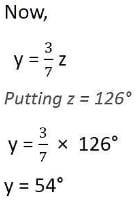Also,  AB║ CD &  MN is transversal. We know that the sum of interior angles on the same side of transversal is supplementry.

x + y = 180°

x + 54° = 180°

x = 180° - 54°

x = 126°

Test: Parallel Lines And Co-Interior Angles - Question 7

Given that AB || CD, intersected by a transversal, if the complement of ∠5 equals the supplement of ∠4, then the measures of ∠4 and ∠5 are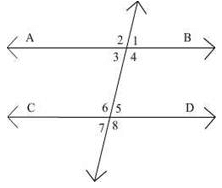Test: Parallel Lines And Co-Interior Angles - Question 8

If a transversal intersects two parallel lines, then the consecutive interior angles are

Test: Parallel Lines And Co-Interior Angles - Question 9

Sum of the measure of an angle and its vertically opposite angle is always.

Test: Parallel Lines And Co-Interior Angles - Question 10

In the figure if AB ||CD, ∠APQ = 50° and ∠PRD = 127° then y is equal to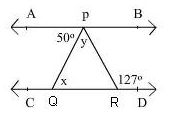Detailed Solution for Test: Parallel Lines And Co-Interior Angles - Question 10
X=50,x+y=127(exterior angle theorem)50+y=127y=127-50y=77
Test: Parallel Lines And Co-Interior Angles - Question 11

In the given figure, the value of y is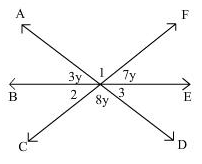Test: Parallel Lines And Co-Interior Angles - Question 12

In the adjoining figure, if PQ || RS, ∠PAB = 45° and ∠ACS = 135°, the value of p and q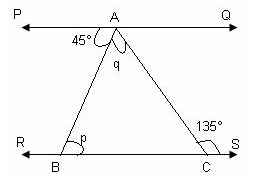Test: Parallel Lines And Co-Interior Angles - Question 13

In the adjoining figure, AB || CD || EF, the value of x is: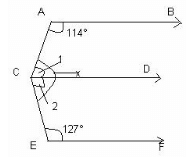Test: Parallel Lines And Co-Interior Angles - Question 14

If two straight lines are perpendicular to a line l, then they are.

Test: Parallel Lines And Co-Interior Angles - Question 15

If two interior angles on the same side of a transversal intersecting two parallel lines in the ratio 2:3 ,then find the larger of two angles.

Detailed Solution for Test: Parallel Lines And Co-Interior Angles - Question 15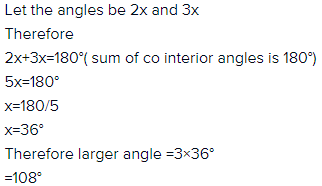## Mathematics (Maths) Class 9

42 videos|378 docs|65 tests
Information about Test: Parallel Lines And Co-Interior Angles Page
In this test you can find the Exam questions for Test: Parallel Lines And Co-Interior Angles solved & explained in the simplest way possible. Besides giving Questions and answers for Test: Parallel Lines And Co-Interior Angles, EduRev gives you an ample number of Online tests for practice

## Mathematics (Maths) Class 9

42 videos|378 docs|65 tests